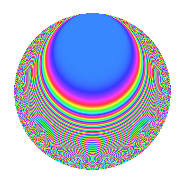# Properties

 Label 403.2.c.bLevel 403 Weight 2 Character orbit 403.c Analytic conductor 3.218 Analytic rank 0 Dimension 32 CM No

# Related objects

## Newspace parameters

 Level: $$N$$ = $$403 = 13 \cdot 31$$ Weight: $$k$$ = $$2$$ Character orbit: $$[\chi]$$ = 403.c (of order $$2$$ and degree $$1$$)

## Newform invariants

 Self dual: No Analytic conductor: $$3.21797120146$$ Analytic rank: $$0$$ Dimension: $$32$$ Sato-Tate group: $\mathrm{SU}(2)[C_{2}]$

## $q$-expansion

The dimension is sufficiently large that we do not compute an algebraic $$q$$-expansion, but we have computed the trace expansion.

 $$\operatorname{Tr}(f)(q) =$$ $$32q - 4q^{3} - 36q^{4} + 20q^{9} + O(q^{10})$$ $$\operatorname{Tr}(f)(q) =$$ $$32q - 4q^{3} - 36q^{4} + 20q^{9} + 4q^{10} - 16q^{12} + 10q^{13} - 16q^{14} + 28q^{16} - 8q^{17} - 16q^{22} - 8q^{23} + 4q^{25} + 18q^{26} + 20q^{27} - 16q^{29} + 40q^{30} - 4q^{35} - 44q^{36} + 12q^{38} + 4q^{39} + 28q^{40} + 28q^{42} - 32q^{43} - 64q^{49} - 64q^{52} - 12q^{53} + 44q^{55} + 8q^{56} + 16q^{61} + 8q^{62} - 76q^{64} - 66q^{65} - 68q^{66} + 64q^{68} + 20q^{69} + 16q^{74} - 32q^{77} - 20q^{78} + 64q^{79} - 16q^{81} + 12q^{82} - 72q^{87} + 80q^{88} + 68q^{90} + 22q^{91} + 28q^{92} + 88q^{94} + 4q^{95} + O(q^{100})$$

## Embeddings

For each embedding $$\iota_m$$ of the coefficient field, the values $$\iota_m(a_n)$$ are shown below.

For more information on an embedded modular form you can click on its label.

Label $$a_{2}$$ $$a_{3}$$ $$a_{4}$$ $$a_{5}$$ $$a_{6}$$ $$a_{7}$$ $$a_{8}$$ $$a_{9}$$ $$a_{10}$$
311.1 2.71381i 0.265781 −5.36475 0.954130i 0.721280i 4.28452i 9.13130i −2.92936 2.58933
311.2 2.67507i −1.67043 −5.15599 2.86564i 4.46851i 5.21058i 8.44248i −0.209666 −7.66579
311.3 2.45635i 2.80039 −4.03363 3.48535i 6.87871i 3.54003i 4.99531i 4.84216 8.56123
311.4 2.44691i 2.35527 −3.98738 2.67528i 5.76313i 0.955539i 4.86294i 2.54728 −6.54617
311.5 2.21678i −2.59178 −2.91410 1.00571i 5.74540i 1.82096i 2.02635i 3.71732 2.22943
311.6 1.99449i −1.58843 −1.97798 2.66789i 3.16810i 1.73927i 0.0439183i −0.476895 −5.32107
311.7 1.88981i −0.327950 −1.57140 1.75790i 0.619765i 0.652358i 0.809976i −2.89245 3.32211
311.8 1.48947i 2.99747 −0.218531 0.692562i 4.46466i 1.15260i 2.65345i 5.98485 1.03155
311.9 1.44935i 0.0382575 −0.100611 3.85977i 0.0554484i 1.66255i 2.75288i −2.99854 5.59415
311.10 1.44015i −2.79139 −0.0740342 2.61757i 4.02002i 4.99660i 2.77368i 4.79185 3.76969
311.11 1.40506i 1.52886 0.0258025 1.01618i 2.14814i 2.90958i 2.84638i −0.662589 −1.42779
311.12 1.01245i −1.05136 0.974950 3.24438i 1.06445i 1.79572i 3.01198i −1.89463 −3.28477
311.13 0.937316i 1.68575 1.12144 0.290760i 1.58008i 3.85549i 2.92577i −0.158249 0.272534
311.14 0.624841i −2.78388 1.60957 0.619834i 1.73948i 2.35216i 2.25541i 4.74999 −0.387298
311.15 0.475674i 0.345654 1.77373 0.161594i 0.164419i 4.07996i 1.79507i −2.88052 −0.0768661
311.16 0.327248i −1.21221 1.89291 2.01769i 0.396693i 0.699230i 1.27395i −1.53055 −0.660284
311.17 0.327248i −1.21221 1.89291 2.01769i 0.396693i 0.699230i 1.27395i −1.53055 −0.660284
311.18 0.475674i 0.345654 1.77373 0.161594i 0.164419i 4.07996i 1.79507i −2.88052 −0.0768661
311.19 0.624841i −2.78388 1.60957 0.619834i 1.73948i 2.35216i 2.25541i 4.74999 −0.387298
311.20 0.937316i 1.68575 1.12144 0.290760i 1.58008i 3.85549i 2.92577i −0.158249 0.272534
See all 32 embeddings
 $$n$$: e.g. 2-40 or 990-1000 Embeddings: e.g. 1-3 or 311.32 Significant digits: Format: Complex embeddings Normalized embeddings Satake parameters Satake angles

## Inner twists

This newform does not have CM; other inner twists have not been computed.

## Hecke kernels

This newform can be constructed as the kernel of the linear operator $$T_{2}^{32} + \cdots$$ acting on $$S_{2}^{\mathrm{new}}(403, [\chi])$$.